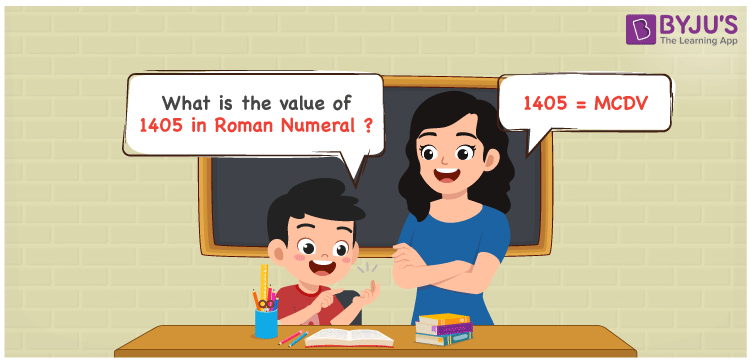# 1405 in Roman Numerals

1405 in Roman Numerals is MCDV. Any Roman numeral uses only the 7 primary symbols which are I, V, X, L, C, D, and M. Here, M represents 1000, C represents 100, D represents 500 and V represents 5. The difference between 500 and 100 can give the number 400 and 1000 and 5 is added to make the sum as 1405. For understanding the rules on Roman Numerals, you can refer to the link Rules to write Roman Numerals

 Number Roman Numeral 1405 MCDV

## How to Write 1405 in Roman Numerals?To convert 1405 into Roman Numerals, we need to represent 1405 with fundamental symbols. 1000 can be represented as M. The representation of 400 in terms of 100 will be against the rule of Roman numerals and hence difference is used. It can be done as 500 – 100. And 5 is represented with the symbol V. Therefore 1405 will be MCDV.

1405 = 1000 + 400 + 5

1405 = 1000 + (500 – 100) + 5

1405 = M + (D – C) + V.

1405 = MCDV

MCDV = 1405.

## Video Lesson on Roman Numerals## Frequently Asked Questions on 1405 in Roman Numerals

### How to write the number 1405 in Roman Numerals?

1405 in Roman Numerals is written as MCDV.

### Do MCDV and IDC represent Roman Numerals?

No. MCDV represents Roman Numerals and it is the number 1405, whereas IDC is an invalid Roman Numeral.

### How to express the number One Thousand Four Hundred and Five in Roman numerals?

The number One Thousand Four Hundred and Five is expressed as MCDV in Roman Numerals.